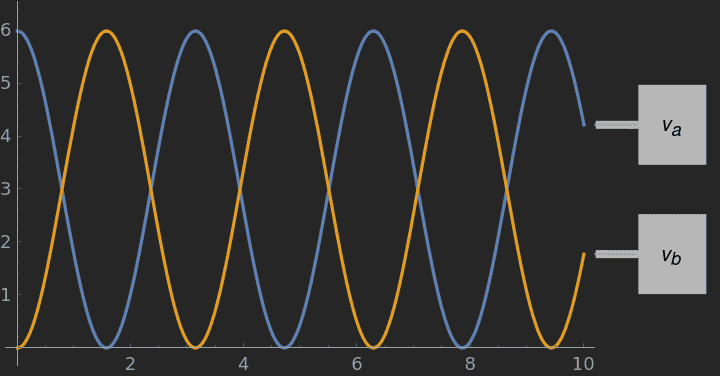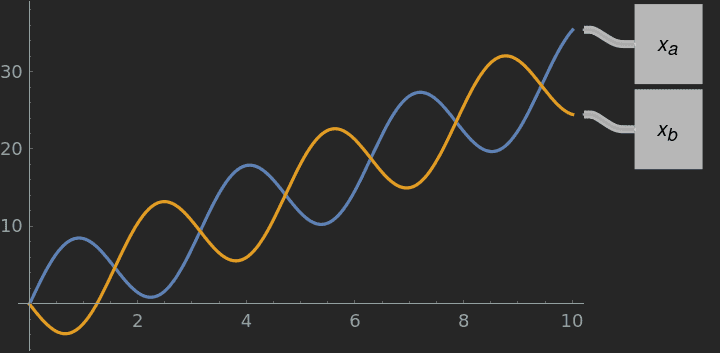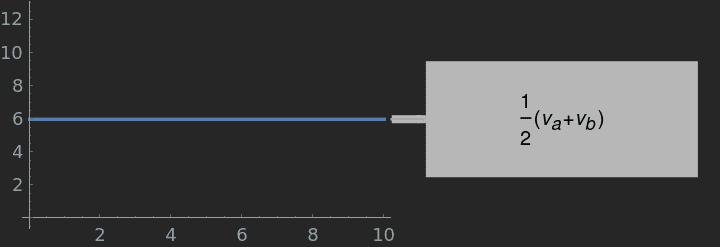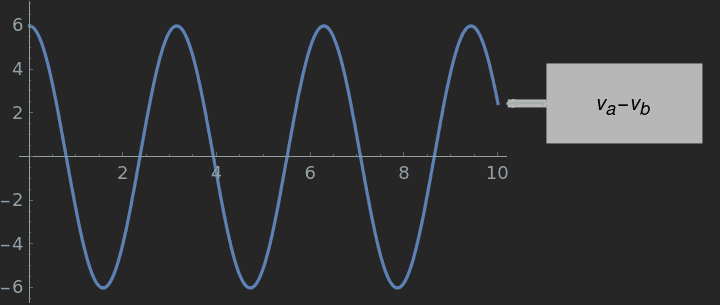# Visualizing the SHM of 2 blocks attached by a spring

yucheng
From Kleppner's Intro to Mechanics (Example 4.7, wording not exact): Two identical blocks a and b each of mass m slide without friction on a straight track. They are attached by a spring with unstretched length l and spring constant k; the mass of the spring is negligible compared to the mass of the blocks. Initially the system is at rest. At t = 0, block a is hit sharply, giving it an instantaneous velocity v 0 to the right. Find the velocity of each block at later times. (Try this yourself if there is a linear air track available—the motion is unexpected.)

After doing some Mathematics, we arrive at the formulas:

Let ##\omega=\sqrt{\frac{k}{m}}##
$$v_{a}=\frac{v_{0}}{2}(1+\cos{\omega t})$$
$$v_{b}=\frac{v_{0}}{2}(1-\cos{\omega t})$$

Question 1: Does anyone have a video/animation that shows such an oscillation? I have tried searching the web but to no avail.

Question 2: Is there a general strategy to visualize such motions based on the equations? Graphs are obviously criptic: just a bunch of lines. Is there a systematic way to understand them?

I have tried using Mathematica to plot the graphs, by fixing arbitrary but still reasonable values for ##v_0=6## and ##\omega=2##:

Graphs of the velocity:Which shows simple harmonic motion as expected.

$$x_a = \frac{6}{2} (t + 2 \sin{2 t})$$
$$x_b = \frac{6}{2} (t - 2 \sin{2 t})$$

The displacement graph? (I got it by integrating the above equations of the velocity)At leats it shows displacement towards the right, presumably more positive y.

Is this method correct? Obviously I am missing out on quite a few details: I am struggling to imagine the actual motion!

Last edited:
•Delta2

Homework Helper
Life becomes a bit more transparent in this case if you look at ##v_a+v_b## and at ##v_a-v_b## :
You 'see' motion of the center of mass and the spring oscillation

•yucheng
yucheng
@BvULast edited: